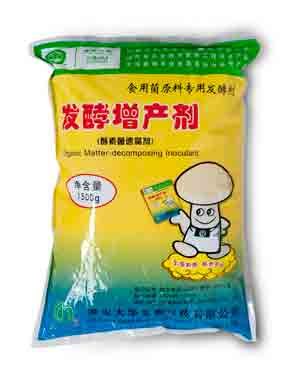• 水产专用
• 大量元素水溶肥料
• 含腐殖酸水溶肥料
• 含氨基酸水溶肥料
• 发酵菌剂
• 沼气发酵菌剂
• 肥水专用
• 净水剂
• 发酵增产剂
• 生物反应堆菌剂
• 秸秆饲料发酵剂
• 酵素菌速腐剂
• 废菌料发酵剂
• 育苗基质
• 水稻育苗基质
• 蓝莓栽培基质
• 蔬菜育苗基质
• 生物有机肥
• 有机肥料
• 生物有机肥
• 食用菌专用
• 发酵增产剂
• 菇宝乐
• 联系我们
• 产品分类
• 发酵菌剂
• 生物反应堆菌剂
• 沼气发酵菌剂
• 肥水专用
• 发酵增产剂
• 秸秆饲料发酵剂
• 废菌料发酵剂
• 酵素菌速腐剂
• 净水剂
• 水溶肥料
• 大量元素水溶肥料
• 含腐殖酸水溶肥料
• 含氨基酸水溶肥料
• 育苗基质
• 蓝莓栽培基质
• 蔬菜育苗基质
• 水稻育苗基质
• 生物有机肥
• 有机肥料
• 生物有机肥
• 水产制剂
• 水产专用
• 食用菌专用
• 菇宝乐
• 发酵增产剂
• 园艺资材
• 有机肥料
• 水稻育苗基质
• 花盆
• 含氨基酸水溶肥料
• 菇宝乐
• 发酵增产剂
• 土壤修复剂
• 土壤调理剂
• 产品分类
• 发酵菌剂
• 水溶肥料
• 育苗基质
• 生物有机肥
• 食用菌专用
• 园艺资材
• 土壤调理剂
• 土壤修复剂
• 水产制剂
• 产品分类
• 土壤调理剂
• 土壤修复剂
• 水产制剂
• 水产专用
• 育苗基质
• 蓝莓栽培基质
• 蔬菜育苗基质
• 水稻育苗基质
• 发酵菌剂
• 发酵增产剂
• 肥水专用
• 沼气发酵菌剂
• 净水剂
• 生物反应堆菌剂
• 秸秆饲料发酵剂
• 废菌料发酵剂
• 酵素菌速腐剂
• 水溶肥料
• 大量元素水溶肥料
• 含氨基酸水溶肥料
• 含腐殖酸水溶肥料
• 生物有机肥
• 有机肥料
• 生物有机肥
• 食用菌专用
• 菇宝乐
• 发酵增产剂
• 园艺资材
• 菇宝乐
• 发酵增产剂
• 花盆
• 水稻育苗基质
• 含氨基酸水溶肥料
• 有机肥料
• 产品分类
• 发酵菌剂
• 发酵增产剂
• 沼气发酵菌剂
• 净水剂
• 生物反应堆菌剂
• 秸秆饲料发酵剂
• 废菌料发酵剂
• 酵素菌速腐剂
• 肥水专用
• 水溶肥料
• 大量元素水溶肥料
• 含腐殖酸水溶肥料
• 含氨基酸水溶肥料
• 育苗基质
• 蓝莓栽培基质
• 蔬菜育苗基质
• 水稻育苗基质
• 生物有机肥
• 有机肥料
• 生物有机肥
• 土壤调理剂
• 食用菌专用
• 菇宝乐
• 发酵增产剂
• 园艺资材
• 有机肥料
• 水稻育苗基质
• 花盆
• 含氨基酸水溶肥料
• 发酵增产剂
• 菇宝乐
• 土壤修复剂
• 水产制剂
• 水产专用
• 产品分类
• 土壤调理剂
• 土壤修复剂
• 水产制剂
• 水产专用
• 发酵菌剂
• 沼气发酵菌剂
• 肥水专用
• 净水剂
• 发酵增产剂
• 生物反应堆菌剂
• 秸秆饲料发酵剂
• 废菌料发酵剂
• 酵素菌速腐剂
• 水溶肥料
• 含氨基酸水溶肥料
• 含腐殖酸水溶肥料
• 大量元素水溶肥料
• 育苗基质
• 蓝莓栽培基质
• 蔬菜育苗基质
• 水稻育苗基质
• 生物有机肥
• 有机肥料
• 生物有机肥
• 食用菌专用
• 菇宝乐
• 发酵增产剂
• 园艺资材
• 含氨基酸水溶肥料
• 菇宝乐
• 发酵增产剂
• 水稻育苗基质
• 有机肥料
• 花盆
• 网站首页
• 关于我们
• 总经理致辞
• 企业文化
• 组织架构
• 公司概况
• 产品分类
• 土壤调理剂
• 水产制剂
• 水产专用
• 园艺资材
• 有机肥料
• 水稻育苗基质
• 花盆
• 含氨基酸水溶肥料
• 菇宝乐
• 发酵增产剂
• 育苗基质
• 蓝莓栽培基质
• 蔬菜育苗基质
• 水稻育苗基质
• 生物有机肥
• 有机肥料
• 生物有机肥
• 食用菌专用
• 发酵增产剂
• 菇宝乐
• 土壤修复剂
• 水溶肥料
• 大量元素水溶肥料
• 含腐殖酸水溶肥料
• 含氨基酸水溶肥料
• 发酵菌剂
• 废菌料发酵剂
• 酵素菌速腐剂
• 沼气发酵菌剂
• 肥水专用
• 净水剂
• 发酵增产剂
• 生物反应堆菌剂
• 秸秆饲料发酵剂
• 产品分类
• 发酵菌剂
• 沼气发酵菌剂
• 肥水专用
• 净水剂
• 发酵增产剂
• 生物反应堆菌剂
• 秸秆饲料发酵剂
• 废菌料发酵剂
• 酵素菌速腐剂
• 水溶肥料
• 大量元素水溶肥料
• 含腐殖酸水溶肥料
• 含氨基酸水溶肥料
• 育苗基质
• 蓝莓栽培基质
• 蔬菜育苗基质
• 水稻育苗基质
• 生物有机肥
• 有机肥料
• 生物有机肥
• 食用菌专用
• 菇宝乐
• 发酵增产剂
• 园艺资材
• 含氨基酸水溶肥料
• 有机肥料
• 花盆
• 菇宝乐
• 发酵增产剂
• 水稻育苗基质
• 土壤调理剂
• 土壤修复剂
• 水产制剂
• 水产专用
• 产品分类
• 园艺资材
• 有机肥料
• 水稻育苗基质
• 花盆
• 含氨基酸水溶肥料
• 菇宝乐
• 发酵增产剂
• 土壤调理剂
• 水产制剂
• 水产专用
• 土壤修复剂
• 发酵菌剂
• 生物反应堆菌剂
• 沼气发酵菌剂
• 肥水专用
• 发酵增产剂
• 秸秆饲料发酵剂
• 废菌料发酵剂
• 酵素菌速腐剂
• 净水剂
• 食用菌专用
• 菇宝乐
• 发酵增产剂
• 水溶肥料
• 大量元素水溶肥料
• 含腐殖酸水溶肥料
• 含氨基酸水溶肥料
• 育苗基质
• 蓝莓栽培基质
• 蔬菜育苗基质
• 水稻育苗基质
• 生物有机肥
• 生物有机肥
• 有机肥料
• 产品分类
• 食用菌专用
• 菇宝乐
• 发酵增产剂
• 水溶肥料
• 大量元素水溶肥料
• 含腐殖酸水溶肥料
• 含氨基酸水溶肥料
• 育苗基质
• 蓝莓栽培基质
• 蔬菜育苗基质
• 水稻育苗基质
• 生物有机肥
• 生物有机肥
• 有机肥料
• 园艺资材
• 有机肥料
• 水稻育苗基质
• 花盆
• 含氨基酸水溶肥料
• 菇宝乐
• 发酵增产剂
• 土壤调理剂
• 土壤修复剂
• 水产制剂
• 水产专用
• 发酵菌剂
• 废菌料发酵剂
• 酵素菌速腐剂
• 发酵增产剂
• 秸秆饲料发酵剂
• 生物反应堆菌剂
• 沼气发酵菌剂
• 肥水专用
• 净水剂
• 产品分类
• 发酵菌剂
• 沼气发酵菌剂
• 肥水专用
• 生物反应堆菌剂
• 净水剂
• 秸秆饲料发酵剂
• 废菌料发酵剂
• 酵素菌速腐剂
• 发酵增产剂
• 土壤修复剂
• 水溶肥料
• 大量元素水溶肥料
• 含腐殖酸水溶肥料
• 含氨基酸水溶肥料
• 育苗基质
• 蓝莓栽培基质
• 蔬菜育苗基质
• 水稻育苗基质
• 水产制剂
• 水产专用
• 食用菌专用
• 菇宝乐
• 发酵增产剂
• 园艺资材
• 有机肥料
• 水稻育苗基质
• 花盆
• 含氨基酸水溶肥料
• 菇宝乐
• 发酵增产剂
• 土壤调理剂
• 生物有机肥
• 生物有机肥
• 有机肥料
• 产品分类
• 育苗基质
• 蓝莓栽培基质
• 蔬菜育苗基质
• 水稻育苗基质
• 生物有机肥
• 有机肥料
• 生物有机肥
• 食用菌专用
• 菇宝乐
• 发酵增产剂
• 土壤调理剂
• 土壤修复剂
• 水产制剂
• 水产专用
• 园艺资材
• 水稻育苗基质
• 有机肥料
• 花盆
• 含氨基酸水溶肥料
• 菇宝乐
• 发酵增产剂
• 发酵菌剂
• 沼气发酵菌剂
• 肥水专用
• 净水剂
• 发酵增产剂
• 生物反应堆菌剂
• 秸秆饲料发酵剂
• 废菌料发酵剂
• 酵素菌速腐剂
• 水溶肥料
• 含腐殖酸水溶肥料
• 大量元素水溶肥料
• 含氨基酸水溶肥料
• 产品分类
• 土壤调理剂
• 土壤修复剂
• 水产制剂
• 水产专用
• 发酵菌剂
• 沼气发酵菌剂
• 肥水专用
• 净水剂
• 发酵增产剂
• 生物反应堆菌剂
• 秸秆饲料发酵剂
• 废菌料发酵剂
• 酵素菌速腐剂
• 水溶肥料
• 含氨基酸水溶肥料
• 含腐殖酸水溶肥料
• 大量元素水溶肥料
• 育苗基质
• 蓝莓栽培基质
• 蔬菜育苗基质
• 水稻育苗基质
• 生物有机肥
• 有机肥料
• 生物有机肥
• 食用菌专用
• 菇宝乐
• 发酵增产剂
• 园艺资材
• 花盆
• 菇宝乐
• 发酵增产剂
• 水稻育苗基质
• 含氨基酸水溶肥料
• 有机肥料
• ### 园艺资材产品名称： 发酵增产剂

发布日期： 2016-08-22

点击数： 1264

询价：

二维码：简述：

### 产品详情

酵增产剂是淮安亚博体育 登录生物科技有限公司引进日本生物技术开发而成的一种新型微生物制剂，内含多种有益菌，高效安全、无任何毒副作用，适用于食用菌栽培原料（如棉籽壳、秸杆、木屑、玉米芯、谷壳、禾草等培养料）的发酵，可用于栽培蘑菇、平菇、草菇、鸡腿菇、姬松茸、大肥菇、秀珍菇、姬菇、金福菇、真姬菇等多种菇类，是生产绿色、无公害食用菌产品的优良制剂。

【作用特点】

发酵增产剂中含有大量的有益微生物群，能产生淀粉酶、蛋白分解酶、纤维分解酶、脂肪分解酶、氧化还原酶等多种催化分解酶，具有极强的催化分解能力，在原料发酵过程中可产生大量的葡萄糖、氨基酸、维生素、酶类等天然活性物质，并且发酵产生的高温能杀灭原料所携带的病原菌、虫卵，而且通过大量有益微生物的拮抗作用，能杀灭或抑制杂菌的繁殖和侵入。

【主要功效】

1、促进养分分解，提高食用菌产量

发酵增产剂用于有机物原料发酵，能将难以分解的植物纤维、木质素等大分子物质催化分解成易被菌丝吸收利用的营养成分，提高原料的有效养分含量。试验表明，使用发酵增产剂发酵原料能促进菌丝生长，出菇期可提前3—5天，且单菇重增加，产量可增加10—30%。

2、增强原料抗杂能力

发酵增产剂在原料发酵过程中，增温迅速，高温持续时间长，可以杀灭原料中携带的霉菌、腐败细菌及虫卵，栽培时不需再添加多菌灵、克霉灵等杀菌剂，另外可以促进栽培料中有益菌的增殖，利用大量有益菌的拮抗作用抑制杂菌生长。

3、减少农药使用，提高产品品质

由于发酵增产剂具有极强的抗杂能力，原料中不必再添加杀菌剂，基本消除了菇体农药残留问题，同时发酵产生的葡萄糖、氨基酸、维生素等多种活性物质，可使菇质鲜嫩，口味纯正，且子实体韧性好，耐贮运，可明显提高产品品质。

上一个：有机肥料

下一个：含氨基酸水溶肥

版权所有：淮安亚博体育 登录生物科技有限公司
技术支持：仕德伟科技 苏ICP备16009131号